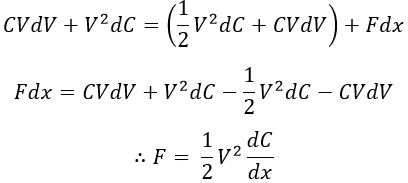# Electrostatic Instrument - Working Principle, Force & Torque Equation

Generally, electrostatic instruments are used as voltmeters only. These instruments work on the principle of static electric field i.e., the electric field produced by stationary charge. Electrostatic instruments have capable of measuring high voltages (>10kV) and can also be used to measure current and power in a circuit with external arrangements.

## Working Principle of Electrostatic Instrument :

The underlying principle of electrostatic instruments is due to force between two oppositely charged bodies between which a potential difference is maintained.

Let us consider two plates to which sufficient potential difference is applied. Now, these two plates get charged with opposite polarity and there exists a force of attraction between them. Suppose, if any plate is made to move. The plate gets moved toward the fixed plate due to the force of attraction. This movement gives rise to the production of deflecting torque, deflecting the pointer attached to it.

But the major problem with electrostatic instruments is, to measure small voltages of the order of a few hundred volts. The distance between the plates should be very less, so as to obtain a minimum amount of force required for the motion. This is due to the fact that the electrostatic forces are very weak and the negligible movements obtained for such small distances cannot be measured.

This difficulty can be overcome up to some extent by using heterostatic connection. But it requires an additional high voltage dc supply, which increases its cost. Another possible way to overcome this difficulty is to step up the voltage being measured.

But, this again increases the cost, and also it is applicable only for alternating voltage measurements. Hence, unless the voltage being measured is sufficiently large, the force is small. Therefore, these instruments are best suited for measuring very high voltages.

## Force and Torque Equation for Electrostatic Instrument :

Consider an electrostatic voltmeter whose plates are charged oppositely by a potential of V volts and the movable plate is connected to a spring shown in the figure below.

Let the force of attraction between the plates be F newton. Due to the force of attraction between the plates, the movable plate moves towards the fixed plate until F balances the restraining force of the spring. Now, the energy E stored by the capacitor (parallel plates) of capacitance C is given by,

#### For a small voltage increment dV in the applied voltage, a small movement dx of the movable plate towards the fixed plate occurs. Due to this voltage variation, a charging current I flow which is given by,We know that the input electrical energy is the product of voltage, current, and time.Now, stored energy for small incremental portion,Therefore, change in stored energy (incremental stored energy - stored energy) is given by,From the law of conservation of energy, we have,Input Energy = Increase in stored energy + Mechanical work doneThe above force equation is for linear electrostatic type instruments. But, in order to derive the force and torque equation for rotational motion as shown in the below figure.

### Disadvantages of Electrostatic Instrument :

#### They can be used for the measurement of voltages alone and that too for high voltage measurement only.Their scale is not uniform since, θ ∝ V2.The cost and size of this instrument are more.They are not robust in construction so, they should be operated carefully.The operating forces are very small when compared to that of electromagnetic instruments.

Do not enter any spam links and messages